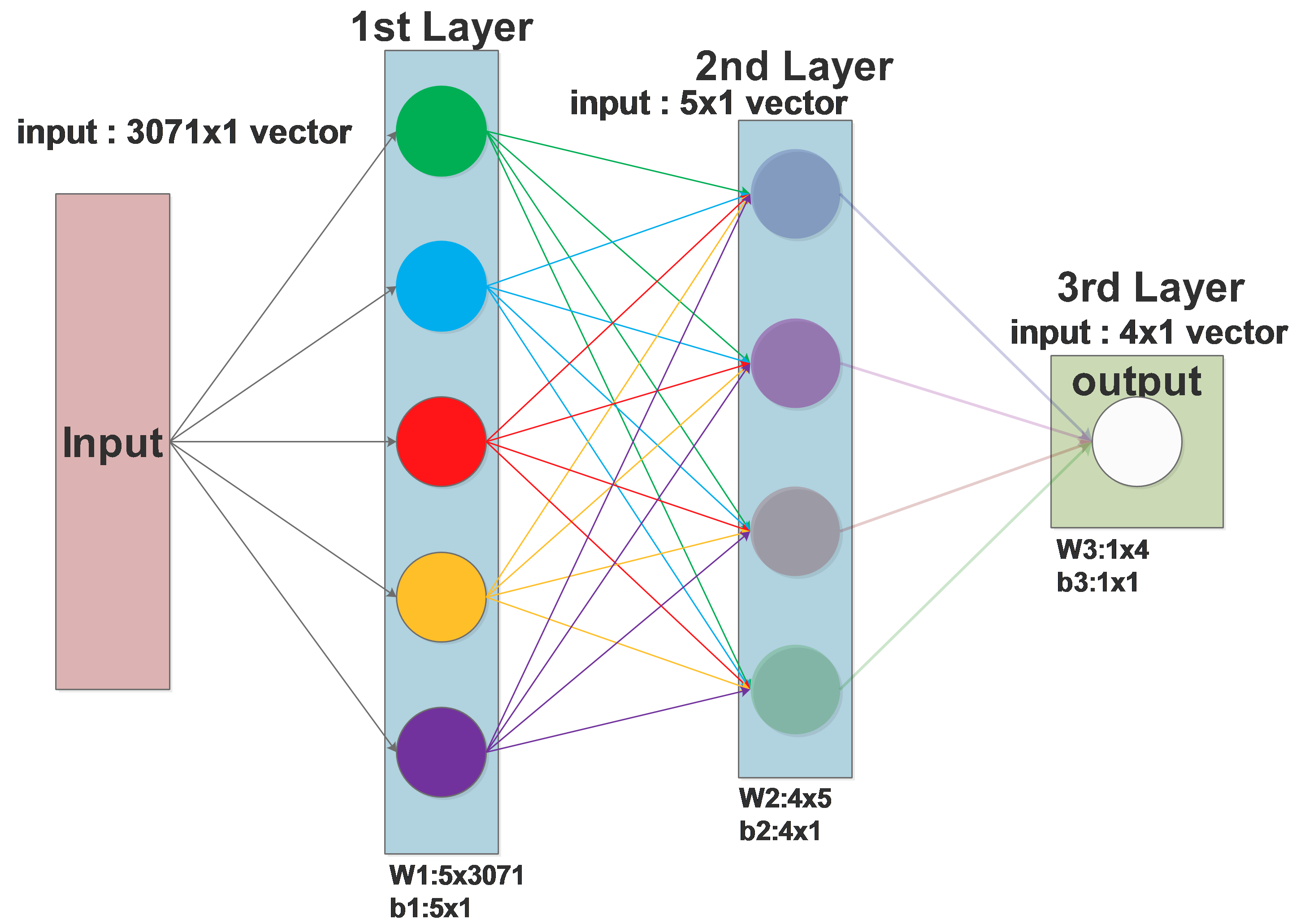# NN Architecture# NN:one neuron

### Simple NeuronThe above diagram shows a neuron in NN, it simulates a real neuron:

it has inputs: $$x_{0},x_{1}\dots x_{i}$$

it has weights for each inputs: $$\omega_{0},\omega_{1}\dots \omega_{i}$$: weight vector

it has bias $$b$$

it has a threshold for the “activation function”

# SVM:multi-class SVM regularization

### Review

For the ith sample $$(x_i,y_i)$$ in the training set, we have the following loss function:

$L_i= \sum_{j≠y_i}max(0,w_j^T\cdot x_i−w_{y_i}^T\cdot x_i+Δ)$

$$w_j^T\cdot x_i$$ is the score classifying $$x_i$$ to class j，and $$w_{y_i}^T\cdot x_i$$ is the score classifying correctly(classify to class $$y_i$$)，$$\omega_i$$ is the $$j$$th row of $$W$$.

### Problem

Problem 1:

Considering the geometrical meaning of the weight vector $$\omega$$, it is easy to find out that $$\omega$$ is not unique, $$\omega$$ can change in a small area and result in the same $$L_i$$.

Problem 2:

It the values in $$\omega$$ is scaled, the loss computed will also be scaled by the same ratio. Considering a loss of 15, if we scale all the weights in $$\omega$$ by 2, the loss will be scaled to 30. But this kind of scaling is meaningless, it doesn’t really represent the loss.

C++ 11 supports multi-threading, for which we previously used pthread or boost. Standard C++ 11’s threading is based on boost::thread, now it’s cross platform and no dependency needed.

#include <thread>
#include <iostream>
{
std::cout << "I am a seperate thread...";
}
void main()
{
//do something else .... //
return;
}


The code is simple, the thread function will run right after the std::thread is declared.

# Effective Modern C++:bind and lambda

### Function binding

std::bind can create a function which is binded to another one:

void originalFunc(std::string a, std::string b, std::string c)
{
std::cout << a << " " << b << " " << c << std::endl;
}//original function, to be binded

void main()
{
//Newly binded function, created by bind function
auto newlyBindedFunc = bind(originalFunc, placeholders::_3, "Fixed", placeholders::_1);
//Call the newly binded function
newlyBindedFunc("New 1st",    "New 2nd",   "New 3rd");
}


# Effective Modern C++:lambda expression

lambda expression can be understood as an anonymous function, it doesn’t need a name, it is created in runtime:

auto lam1 = []()
{
cout<<"Hello World!";
};
lam1();


The code above shows a simplest lambda expression, when lam1() is called, it will print “Hello World!”.

### How is the lambda expression existed in C++?

It is existed a closure class, each lambda is a unique closer class generated by the compiler in runtime. So it is a object and can be copied:

# Effective Modern C++:shared_ptr

shared_ptr is a pointer allowed to be copied, it is like the normal pointer we use, but it’s smart for its counting function.

A normal pointer is not safe as when we are deleting it, we don’t know if someone else is using it. The shared_ptr count the users of this pointer, when someone release the pointer, its count will decrement, the memory block where it points will release only when the count becomes 0, which means no one is using it.

Initialize a shared_ptr:

shared_ptr<int> s{ new int{3} };


# Effective Modern C++:unique_ptr

You should include when using smart pointers

### unique_ptr

Just as its name means, unique_ptr guarantees that there is only one pointer point to a specific memory block. So it cannot be copied by only moved.

Init a unique_ptr

unique_ptr<int> p{ new int };
unique_ptr<int> p{ new int{4} };


As you can see, smart pointers are wraps of normal pointers, we call them “raw” pointers.